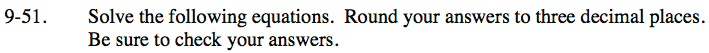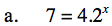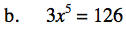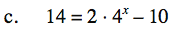### Home > INT3 > Chapter 9 > Lesson 9.1.4 > Problem9-51

9-51.
1. Solve the following equations. Round your answers to three decimal places.

1. 7 = 4.2x

2. 3x5 = 126

3. 14 = 2 · 4x – 10Take the log of both sides of the equation.Isolate the x term on one side of the equation.

x ≈ 2.112"Undo" the operations furthest away from x before the operations closer to it.